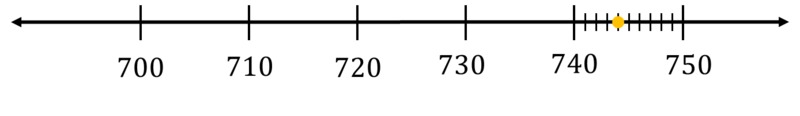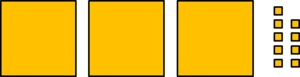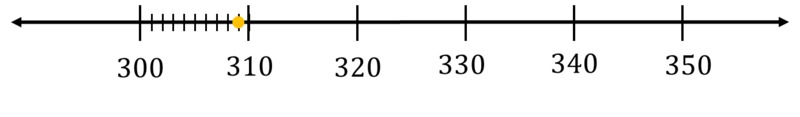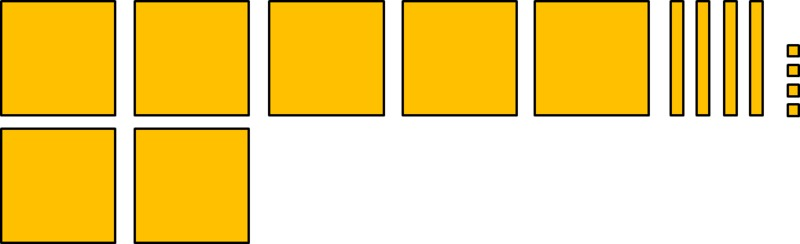# Looking at Numbers Every Which Way

Alignments to Content Standards: 2.NBT.A.1 2.NBT.A.3

1. 127 is a number.
• Write it as a sum of 100's, 10's, and 1's.
• Write its name in words.
• Draw a picture to represent the number.
• Locate it on the number line.
2. 500+60+8 is a number.
• Write it as a three-digit number.
• Write its name in words.
• Draw a picture to represent the number.
• Locate it on the number line.
3. Six hundred and nine is a number.
• Write it as a three-digit number.
• Write it as a sum of 100's, 10's, and 1's.
• Draw a picture to represent the number.
• Locate it on the number line.
4. The picture represents a number. The big square represents 100, the rectangle represents 10, and the small square represents 1.• Write it as a three-digit number.
• Write it as a sum of 100's, 10's, and 1's.
• Write its name in words.
• Locate it on the number line.
5. A number is shown on the number line.• Write it as a three-digit number.
• Write it as a sum of 100's, 10's, and 1's.
• Write its name in words.
• Draw a picture to represent the number.

## IM Commentary

This task gives students the opportunity to work with multiple representations of base-ten numbers. The standard 2.NBT.3 asks students to read and write numbers to 1000 using base-ten numerals, number names, and expanded form. This task addresses all of these and extends it by asking students to represent the numbers with pictures and on the number line, which supports the understanding described in 2.NBT.1. Students who are still grappling with the meaning of base-ten numerals might benefit from having base-ten blocks on hand. Attached is a black line master for a place-value mat which can help scaffold students who are having trouble. Eventually, students should be able to do this task without concrete representations, however.

## Solution

1. 127 = 100 + 20 + 7. This number is one hundred and twenty-seven. Here is a picture (it is a rough sketch based on the picture above without the details for the hundreds and tens):Here it is on the number line:2. 500+60+8 = 568. The number is five hundred sixty-eight. Here is a picture:Here it is on the number line:3. 309 = 300 + 9. Here is a picture:Here is is on the number line:4. 240 = 200+40. This number is two hundred forty. Here it is on the number line:5. 744 = 700 + 40 + 4. This number is seven hundred forty-four. Here is a picture of it: